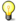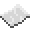# math.cosNote: The argument must be given in radians. If it is in degrees, use the math.rad function to convert it.ExamplePrint the cosine of user input
Read a line from the user, convert it to radians, then print its cosine.
Code
```<nowiki>
</nowiki>
```
Output Depends on what the user wrote. For instance, if they were to enter `90`, the number printed would be `-1`.

math.cos
Function
Syntax
math.cos(
• num : number
)

Returns number
API math
Source Lua (source)

Return the cosine of num.